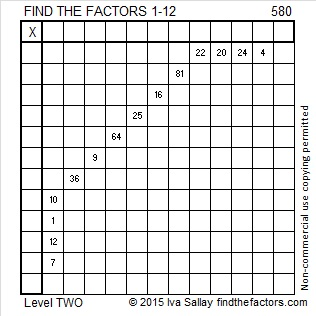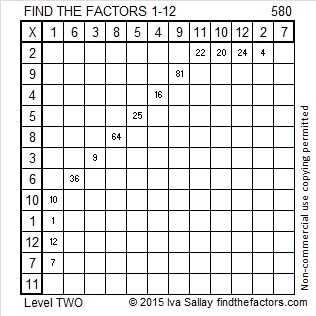# 580 and Level 2

580 is the sum of all the prime numbers from 83 to 107. Do you know what those consecutive prime numbers are?

580 is also the hypotenuse of four Pythagorean triples. In each case can you find the factor of 580 that is the greatest common factor of the triple?

• 68-576-580
• 96-572-580
• 348-464-580
• 400-420-580Print the puzzles or type the solution on this excel file: 12 Factors 2015-08-10

—————————————————————————————————

• 580 is a composite number.
• Prime factorization: 580 = 2 x 2 x 5 x 29, which can be written 580 = (2^2) x 5 x 29
• The exponents in the prime factorization are 2, 1, and 1. Adding one to each and multiplying we get (2 + 1)(1 + 1)(1 + 1) = 3 x 2 x 2 = 12. Therefore 580 has exactly 12 factors.
• Factors of 580: 1, 2, 4, 5, 10, 20, 29, 58, 116, 145, 290, 580
• Factor pairs: 580 = 1 x 580, 2 x 290, 4 x 145, 5 x 116, 10 x 58, or 20 x 29
• Taking the factor pair with the largest square number factor, we get √580 = (√4)(√145) = 2√145 ≈ 24.083189—————————————————————————————————